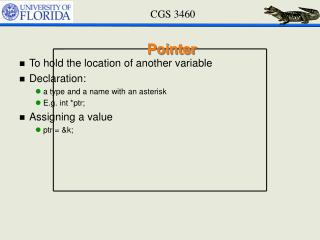DownloadDownload PresentationPointer

# Pointer

Télécharger la présentation## Pointer

- - - - - - - - - - - - - - - - - - - - - - - - - - - E N D - - - - - - - - - - - - - - - - - - - - - - - - - - -
##### Presentation Transcript

1. Pointer • To hold the location of another variable • Declaration: • a type and a name with an asterisk • E.g. int *ptr; • Assigning a value • ptr = &k;

2. Pointers and Structures • Declaration struct date *datePtr; • Initialization datePtr = &today; • Access members • (*datePtr).year • datePtr->year • struct date{ • int day; • char month; • int year; • }; • struct date today;

3. Structures contains pointers • Pointers can be members in a structure struct date{ int day; char* month; int year; }; • Special case: struct date{ int day; char* month; int year; struct date *next; };

4. Linked List • What is a list? • Node • Link • An alternative to Array • Pros/Cons • + More flexible • + Can grow and shrink as necessary • + Insert and delete node with particular index • - Cannot be randomly accessed • + sorted (ordered list)

5. Linked List • Operations • Start a list • Create a node • Insert • Delete • Search • Print

6. Memory Allocation • malloc is by far most frequently used • Defined in <stdlib.h> void *malloc(size_t size); • Allocate memory space with size bytes • Why does it return a void pointer? • Because it doesn't matter to malloc to what type this memory will be used for • If no memory of the size available, will return null • Be sure to check whether it is null;

7. Function sizeof • Helpful when dynamically allocating memory • sizeof(data type) returns a size_t of the data type in byte(s) • For a typical 32-bit machine • sizeof(int) returns 4

8. Free Allocated Space • Very important • System won’t automatically take back memory space allocated through malloc • If not free, a memory leak • How? • Use function • void free(void *ptr); • It release the memory space referenced by ptr • Note that free can take in NULL, as specified by ANSI

9. Build a Linked List • Build a structure representing a node struct studentRecord { int idNum; struct studentRecord *next; }; • Initialize the node struct studentRecord *first = NULL; NULL first

10. Create a node • Follow these steps: • Allocate memory for the node • Set data into the node struct studentRecord *new_student; new_student = malloc(sizeof(struct studentRecord )); (* new_student).idNum = 1; new_student next = NULL; new_student

11. Insert a node: insert in the front(empty) • Follow these steps: • Create a node • Set the node pointing to the front of the list • Set it as the starting node of this list new_student = malloc(sizeof(struct studentRecord )); new_student  idNum = 2; new_student next = first; first = new_student ; NULL new_student first

12. Insert a node: insert in the front(not empty) • Follow these steps: • Create a node • Set the node pointing to the front of the list • Set it as the starting node of this list new_student = malloc(sizeof(struct studentRecord )); new_student  idNum = 2; new_student next = first; first = new_student ; NULL first new_student

13. Insert a node: insert in middle • To insert a new node after node called pt, follow these steps: • Create a node • Set the node pointing to the next node after pt in the list • Set it as the next node of this list after pt new_student = malloc(sizeof(struct studentRecord )); new_student  idNum = 2; new_student next = pt  next; pt  next = new_student ; first pt NULL new_student

14. Traversing along the List for (p = first; p != NULL; p = pnext) { …. } int Length(struct studentRecord* first) { int count = 0; struct studentRecord* current = first; while (current != NULL) { count++; current=current->next; } return(count); }

15. Get nth Element in List int GetNth(struct studentRecord * first, int index) { struct studentRecord * current = first; int count = 0; // the index of the node while (current != NULL) { if (count == index) return(current idNum ); count++; current = current  next; } return(-1); // if we get to this line, the caller was asking // for a non-existent element }

16. Delete • Delete (almost reverse of insertion) • locating the node to be deleted (see search a linked list) • altering the previous node to bypass the deleted node • calling free to reclaim the space occupied by the deleted node

17. Example for deleting • To delete a node p from a linked list, you should also know the node which is in front of p in the list (q) if (p != NULL) { q  next = p  next; free(p); } q p NULL first

18. Example for deleting • If deleting the first node in the list if( p == first ) { first = p->next; free(p); } p NULL first